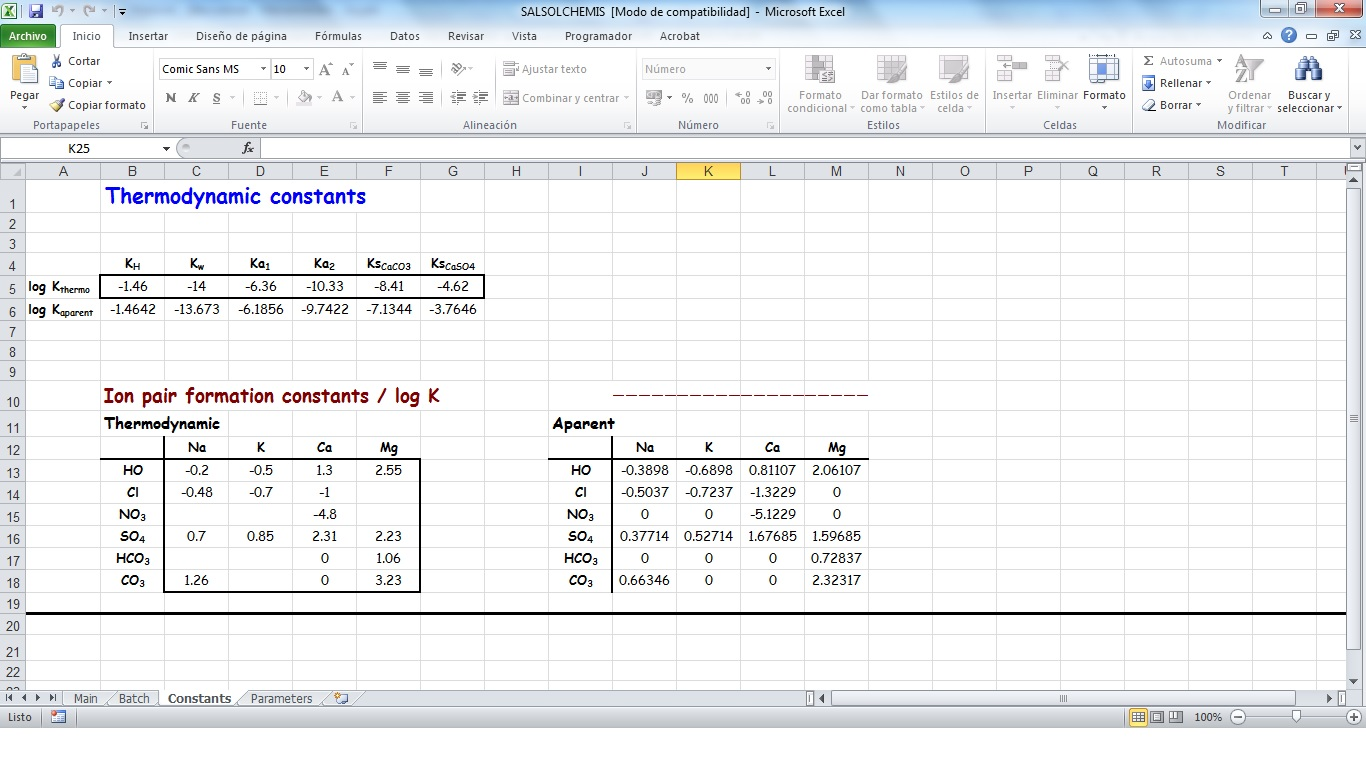# SALSOLCHEMIS: a spreadsheet application to calculate the ionic speciation of saline soil solutions and irrigation waters

SALSOLCHEMIS (SALine SOLution CHEMistry Ionic Speciation) is a computer program developed to calculate the equilibrium properties of saline, calcareous and gypsiferous soil solutions and irrigation waters at 25ºC, pH between 3 and 11 and electrical conductivity (EC25) lower than 20 dS m-1. SALSOLCHEMIS is an ion-association model for the ions sodium, potassium, magnesium, calcium, chloride, nitrate, sulphate, bicarbonate and carbonate. SALSOLCHEMIS is able to carry out calculations of ionic speciation, ionic activities, ionic activity products, and EC25. SALSOLCHEMIS is written in Microsoft Visual Basic© and developed as a spreadsheet application. Input data to the model are entered in the sheets of a Microsoft Excel© workbook, from which SALSOLCHEMIS is called. Following calculations output data are written in the same workbook.

The essential steps for running the SALTIRSOIL model are described below.

SALSOLCHEMIS is copyrighted software by the author, distributed free for educational and research purposes and downloadable here.

The SALTIRSOIL_M model has featured several research articles which can be accessed from this page.### Main sheet

This is the sheet from where the application SALSOLCHEMIS.exe is called to process both individual and multiple systems in a batch, and where the equilibrium results are written for individual systems. To do this the main ion composition of your aqueous system has to be typed in the framed cells of row 5 in this sheet under the heading "Total ion concentrations in the aqueous system in mmol/L". Next once SALSOLCHEMIS is called by pressing the button "Calculate" it carries out the calculations and writes the concentrations of ions, ion pairs, the EC25, ion activity coefficients, pCO2 and ionic activity products.### Batch sheet

Usually one has more than one system to process, often lots of them. This is the sheet where the input and output data of multiple systems are written. The first row is left for the column headings. Then the analytical input data are entered in the second and subsequent rows. When we are done with all the input data, we turn to the "Main" sheet and press the button "Calculate". When SALSOLCHEMIS finishes the calculations, writes the results for every solution in its corresponding row. Every aqueous system has to be labelled with some code significant for the user, which is introduced in the first column.### Constants sheet

The values of the thermodynamic constants used by SALSOLCHEMIS are entered in this sheet. In row 5 there are several framed cells with the base-10 logarithm of the following constants: Henry solubility constant of carbon dioxide in water (KH), the constant of water ionization (Kw), the first and second ionization constants of the carbonic acid (Ka1 and Ka2), and as supplemental information the solubility products of calcite and gypsum. In the framed matrix to the left of the sheet, we have the base-10 logarithm of the ion pair formation constants. An empty cell or containing a zero means that the ion pair does not form.### Parameters sheet

The values of the parameters used by SALSOLCHEMIS to calculate the electrical conductivity at 25ºC (EC25) are entered in this sheet. This sheet is arranged in four frames: the top frame for the charge, ionic mobility and molar mass of free ions and neutral species, the second and third frames for the electrical charge and equivalent ionic mobility for each one of the ion pairs that can be formed by combination of the previous free ions, and finally the bottom frame for the coefficients of the empirical equation used to calculate the electrical conductivity, the intercept in cell E19 and the slope in cell E20.

### Let's get started with SALSOLCHEMIS following these two steps:

1. Click on the button below and and save the compressed zip file in your computer.
2. Extract and save its content wherever you want.### Related publications:

1. Calcite and gypsum solubility products in water-saturated salt-affected soil samples at 25 ºC and at least up to 14 dS m-1 (2010). European Journal of Soil Science 61(2), pp. 255-270. doi: 10.1111/j.1365-2389.2009.01214.x
2. An empirical equation to calculate soil solution electrical conductivity at 25 ºC from major ion concentrations (2010). European Journal of Soil Science 61(6), pp. 980-993. doi: 10.1111/j.1365-2389.2010.01284.x